# Count of triangles

Given a square ABCD and on each side 10 internal points.

Determine the number of triangles with vertices at these points.

x =  9400

### Step-by-step explanation: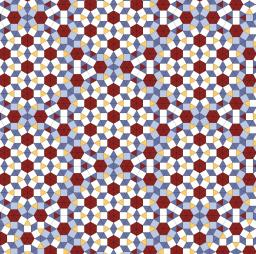Did you find an error or inaccuracy? Feel free to write us. Thank you!Tips to related online calculators
Would you like to compute count of combinations?

#### You need to know the following knowledge to solve this word math problem:

We encourage you to watch this tutorial video on this math problem:

## Related math problems and questions:

• Combi-triangle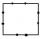On each side of the square is marked 10 different points outside the vertices of the square. How many triangles can be constructed from this set of points, where each vertex of the triangle lie on the other side of the square?
• N points on the sideThere is an equilateral triangle A, B, C on each of its inner sides lies N=13 points. Find the number of all triangles whose vertices lie at given points on different sides.
• Square 2Points D[10,-8] and B[4,5] are opposed vertices of the square ABCD. Calculate area of the square ABCD.
• VariationsDetermine the number of items when the count of variations of fourth class without repeating is 42 times larger than the count of variations of third class without repetition.
• SquarePoints A[9,9] and B[-4,1] are adjacent vertices of the square ABCD. Calculate the area of the square ABCD.
• Points in planeThe plane is given 12 points, 5 of which is located on a straight line. How many different lines could by draw from this points?
• Circle and squareAn ABCD square with a side length of 100 mm is given. Calculate the radius of the circle that passes through the vertices B, C and the center of the side AD.
• Combinatorics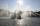The city has 7 fountains. Works only 6. How many options are there that can squirt ?
• Internal anglesThe ABCD is an isosceles trapezoid, which holds: |AB| = 2 |BC| = 2 |CD| = 2 |DA|: On its side BC is a K point such that |BK| = 2 |KC|, on its side CD is the point L such that |CL| = 2 |LD|, and on its side DA the point M is such that | DM | = 2 |MA|. Dete
• Recursion squaresIn the square, ABCD has inscribed a square so that its vertices lie at the centers of the sides of the square ABCD. The procedure of inscribing the square is repeated this way. The side length of the square ABCD is a = 22 cm. Calculate: a) the sum of peri
• Larger perimeter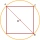There are a square and a circle that passes through two adjacent vertices of the square (end points of side a) and the center of the opposite side (c). Which of the plane shape has a larger perimeter?There are 15 boys and 12 girls at the graduation party. Determine how many four couples can be selected.Show that the points P1 (5,0), P2 (2,1) & P3 (4,7) are the vertices of a right triangle.How many ways can be rewarded 9 participants with the first, second, and third prizes in a sports competition?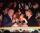At the table sit 10 people, 5 on one side and 5 on the other side. Among them are 3 pairs. Every pair wants to sit opposite each other. How many ways can they sit?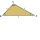Five sticks with a length of 2,3,4,5,6 cm. How many ways can you choose three sticks to form three sides of a triangle?Show that the points D(2,1), E(4,0), F(5,7) are vertices of a right triangle.